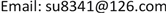1中国人民大学附属中学，北京

2中国科学院物理研究所，北京1. 前言

2. 施密特正交化与张量概念的引入

e 1 = e 2 × e 3 e 1 ⋅ ( e 2 × e 3 )

e 2 = e 3 × e 1 e 1 ⋅ ( e 2 × e 3 )

e 3 = e 1 × e 2 e 1 ⋅ ( e 2 × e 3 )

k = a e 1 + b e 2 + c e 3 (1)

k ⋅ e 1 = a e 1 ⋅ e 1 = a

k ⋅ e 2 = b e 2 ⋅ e 2 = b

k ⋅ e 3 = c e 3 ⋅ e 3 = c

k = ( k ⋅ e 1 ) e 1 + ( k ⋅ e 2 ) e 2 + ( k ⋅ e 3 ) e 3

⇒ k = k 1 e 1 + k 2 e 2 + k 3 e 3 (2)

k = k i e i (3)

e ′ i = ( e ′ i ⋅ e j ) e j = β i j e j (4)

x ′ i = ( x j e j ) ⋅ e ′ i = ( e ′ i ⋅ e j ) x j = β i j x j (5)

(其中 β i j = e ′ i ⋅ e j )

e ′ i = ( e ′ i ⋅ e j ) e j = ( β − 1 ) j i e j (6)

x ′ i = ( x j e j ) ⋅ e ′ i = ( e ′ i ⋅ e j ) x j = ( β − 1 ) j i x j (7)

r = r ( y 1 , y 2 , y 3 ) = y i ε i

r = r ( y 1 , y 2 , y 3 ) = x i e i

d r = ∂ r ∂ y i d y i = ( ∂ x j ∂ y i e j ) d y i

ε i = ∂ r ∂ y i = ∂ x j ∂ y i e j (8)

ε i = ∇ y i = ∂ y i ∂ x j e j (9)

ε i ⋅ ε k = ∂ x j ∂ y i e j ⋅ ∂ y k ∂ x l e l = ∂ x j ∂ y i ∂ y k ∂ x l e j ⋅ e l = ∂ x j ∂ y i ∂ y k ∂ x l δ j l = ∂ x j ∂ y i ∂ y k ∂ x j = δ i k

ε i = ∂ r ∂ y i ， ε i = ∂ r ∂ y i (10)

1) 零阶张量：零阶张量有 n 0 = 1 个元素且其数值与坐标变换无关，即： ϕ ′ = ϕ 。例如基础物理中的时间t，其不随坐标变换而发生变化。

2) 一阶张量：一阶张量有 n 1 = n 个元素，在坐标变换下分为一阶协变张量与一阶逆变张量

3) 二阶张量：二阶张量有 n 2 个元素，在坐标变换下分为二阶协变张量，二阶逆变张量与二阶混合张量。

4) 高阶张量：有 n p + q 个元素，在一般物理理论之中不常用，故这里从略。

C i 1 i 2 ⋯ i p j 1 j 2 ⋯ j p = A i 1 i 2 ⋯ i p j 1 j 2 ⋯ j p ± B i 1 i 2 ⋯ i p j 1 j 2 ⋯ j p

∵ ε i = ε i ( ε j ⋅ ε j ) = ( ε i ⋅ ε j ) ε j = g i j ε j

ε i = ε i ( ε j ⋅ ε j ) = ( ε i ⋅ ε j ) ε j = g i j ε j

A i = A ⋅ ε i = g i j A ⋅ ε j = g i j A j

A i = A ⋅ ε i = g i j A ⋅ ε j = g i j A j

∴ 度规张量 g i j 可以用于协变与逆变分量的相互转化。这一点并不奇怪，从度规的定义上来看我们可以形象的认为度规是刻画两个空间几何关系的一个张量，所以当然可以将协变量“换算”为逆变量。

A ⋅ B = A i ε i ⋅ B j ε j = A i B j g i j = A i B j (11)

3. 结论